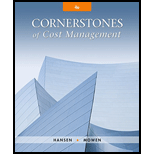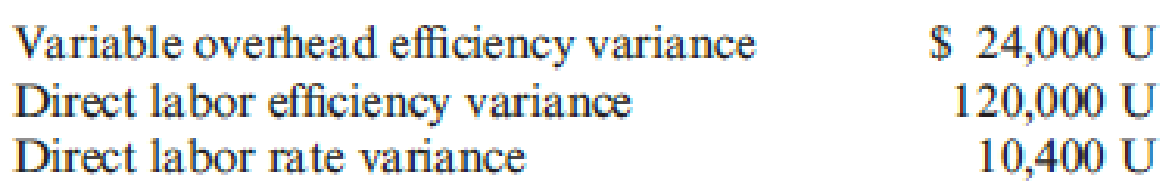# Misterio Company uses a standard costing system. During the past quarter, the following variances were computed: Misterio applies variable overhead using a standard rate of $2 per direct labor hour allowed. Two direct labor hours are allowed per unit produced. (Only one type of product is manufactured.) During the quarter, Misterio used 30 percent more direct labor hours than should have been used. Required: 1. What were the actual direct labor hours worked? The total hours allowed? 2. What is the standard hourly rate for direct labor? The actual hourly rate? 3. How many actual units were produced?BuyFindarrow_forward ### Cornerstones of Cost Management (C... 4th Edition Don R. Hansen + 1 other Publisher: Cengage Learning ISBN: 9781305970663 #### Solutions Chapter SectionBuyFindarrow_forward ### Cornerstones of Cost Management (C... 4th Edition Don R. Hansen + 1 other Publisher: Cengage Learning ISBN: 9781305970663 Chapter 9, Problem 31P Textbook Problem 246 views ## Misterio Company uses a standard costing system. During the past quarter, the following variances were computed:Misterio applies variable overhead using a standard rate of$2 per direct labor hour allowed. Two direct labor hours are allowed per unit produced. (Only one type of product is manufactured.) During the quarter, Misterio used 30 percent more direct labor hours than should have been used.Required: 1. What were the actual direct labor hours worked? The total hours allowed? 2. What is the standard hourly rate for direct labor? The actual hourly rate? 3. How many actual units were produced?

1.

To determine

Calculate the actual direct labor hours worked and the total hours allowed.

### Explanation of Solution

Calculate the actual direct labor hours worked:

Use variable overhead efficiency variance formula to calculate actual direct labor hour.

Step 1: Calculate the standard hours.

Variable overheadefficiency variance} = [(AHSH)×SVOR]$24,000 = [(1.3 SHSH)×$2]$24,000 = [0.3SH×$2]$24,000 = 0.6 SHSH=($24,0000

2.

To determine

Calculate the standard hour rate for direct labor and the actual hour rate.

3.

To determine

Calculate the number of actual units produced.

### Still sussing out bartleby?

Check out a sample textbook solution.

See a sample solution

#### The Solution to Your Study Problems

Bartleby provides explanations to thousands of textbook problems written by our experts, many with advanced degrees!

Get Started

Find more solutions based on key concepts
What is a VAN?

Accounting Information Systems

DISCOUNTED PAYBACK Refer to Problem 11-1. What is the projects discounted payback?

Fundamentals of Financial Management, Concise Edition (with Thomson ONE - Business School Edition, 1 term (6 months) Printed Access Card) (MindTap Course List)

Explain the concept of vesting.

PAYROLL ACCT.,2019 ED.(LL)-TEXT